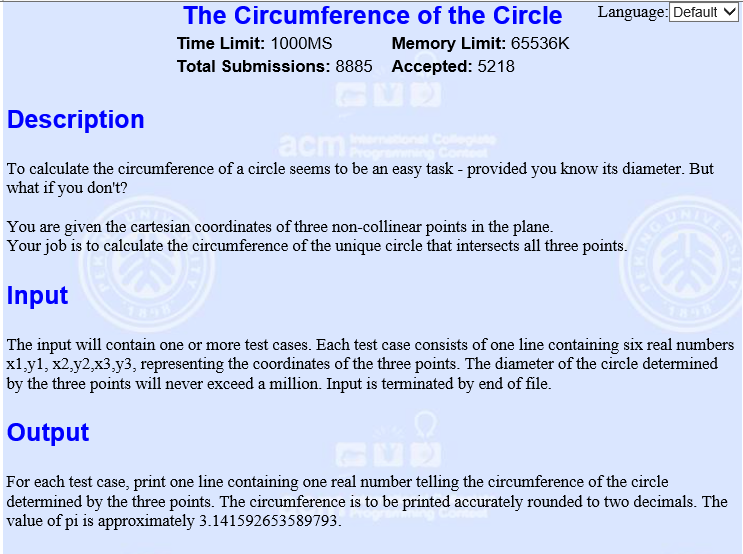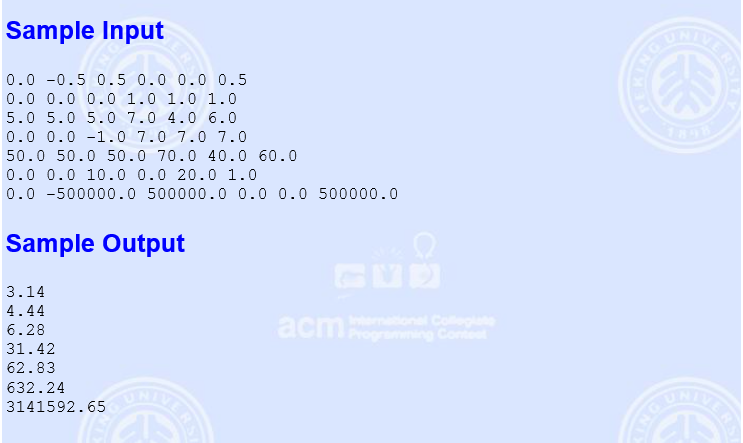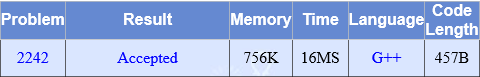• 给出不共线的3点坐标，求所确定的周长。#include <iostream>
#include <cmath>
#include <iomanip>
using namespace std;
//抄博友程序  海伦公式  三角形外接圆半径公式 背
int main()
{
double x1,y1,x2,y2,x3,y3,a,b,c,p,s,r;
while(cin>>x1>>y1>>x2>>y2>>x3>>y3)
{
a=sqrt((x1-x2)*(x1-x2)+(y1-y2)*(y1-y2));
b=sqrt((x2-x3)*(x2-x3)+(y2-y3)*(y2-y3));
c=sqrt((x3-x1)*(x3-x1)+(y3-y1)*(y3-y1));
p=(a+b+c)/2;
s=sqrt(p*(p-a)*(p-b)*(p-c));
r=(a*b*c)/(4*s);
cout<<fixed<<setprecision(2)<<2.0*r*3.14159265358979<<endl;
}
}


展开全文• 已知三角形面积可以由三角形外接圆半径公式求出外接圆的半径： 由正弦定理，a/sinA=b/sinB=c/sinC=2R,得sinA=a/(2R),又三角形面积公式Ｓ=(bcsinA)/2,所以S=(abc)/(4R),故R=(abc)/(4S). 然后即可求出圆的周长 ...
The Circumference of the Circle
Time Limit: 1 Second      Memory Limit: 32768 KB
To calculate the circumference of a circle seems to be an easy task - provided you know its diameter. But what if you don't?
You are given the cartesian coordinates of three non-collinear points in the plane.Your job is to calculate the circumference of the unique circle that intersects all three points.
Input Specification
The input file will contain one or more test cases. Each test case consists of one line containing six real numbers x1,y1, x2,y2,x3,y3, representing the coordinates of the three points. The diameter of the circle determined by the three points will never exceed a million. Input is terminated by end of file.
Output Specification
For each test case, print one line containing one real number telling the circumference of the circle determined by the three points. The circumference is to be printed accurately rounded to two decimals. The value of pi is approximately 3.141592653589793.
Sample Input
0.0 -0.5 0.5 0.0 0.0 0.5
0.0 0.0 0.0 1.0 1.0 1.0
5.0 5.0 5.0 7.0 4.0 6.0
0.0 0.0 -1.0 7.0 7.0 7.0
50.0 50.0 50.0 70.0 40.0 60.0
0.0 0.0 10.0 0.0 20.0 1.0
0.0 -500000.0 500000.0 0.0 0.0 500000.0

Sample Output
3.14
4.44
6.28
31.42
62.83
632.24
3141592.65

给出圆上的三点求圆的周长，给出的三点构成圆的内接三角形，可以求出三角形三边的长度，由海伦公式可以求出三角形面积：
已知三角形三边a,b,c，则 　　p=(a+b+c)/2　　S=√[p(p-a)(p-b)(p-c)]
已知三角形面积可以由三角形外接圆半径公式求出外接圆的半径：
由正弦定理，a/sinA=b/sinB=c/sinC=2R,得sinA=a/(2R),又三角形面积公式Ｓ=(bcsinA)/2,所以S=(abc)/(4R),故R=(abc)/(4S).
然后即可求出圆的周长

#include <iostream>
#include <cmath>
#include <iomanip>
using namespace std;
const double pi=3.141592653589793;
int main()
{
double x1,y1,x2,y2,x3,y3,a,b,c,st,cir,r,p;
while(cin>>x1>>y1>>x2>>y2>>x3>>y3)
{
a=sqrt((x1-x2)*(x1-x2)+(y1-y2)*(y1-y2));
b=sqrt((x1-x3)*(x1-x3)+(y1-y3)*(y1-y3));
c=sqrt((x3-x2)*(x3-x2)+(y3-y2)*(y3-y2));

p=(a+b+c)/2;

st=sqrt(p*(p-a)*(p-b)*(p-c));//三角形面积，海伦公式

r=a*b*c/(4*st);

cir=2*pi*r;

cout.setf(ios::fixed);
cout.precision(2);
cout<<cir<<endl;
}
return 0;
}

cout.setf(ios::fixed);不设置为定点小数的话，cout.precision(2)指的是有效位数为2，如3.1；
设置为定点小数后，cout.precision(2)指的是小数有效位数为2，如3.14


展开全文input each output ios file
• 三角形三边为 a、b、c 半周长 p=(a+b+c)/2 三角形面积 S=√[p(p-a)(p-b)(p-c)]   内切圆半径 r = S/p=√[(p-a)(p-b)(p-c)/p]= ½√...外接圆半径 R= abc/(4S)= ¼ abc/√[p(p-a)(p-b)(p-c)]= abc/√[(a+b+c)(-a+b+
设三角形三边为 a、b、c
半周长 p=(a+b+c)/2
三角形面积 S=√[p(p-a)(p-b)(p-c)] ……（海伦公式）
内切圆半径 r = S/p=√[(p-a)(p-b)(p-c)/p]= ½√[(-a+b+c)(a-b+c)(a+b-c)/(a+b+c)]
外接圆半径 R= abc/(4S)= ¼ abc/√[p(p-a)(p-b)(p-c)]= abc/√[(a+b+c)(-a+b+c)(a-b+c)(a+b-c)]
R、r、S 关系rR = S/p * abc/(4S) = abc/[2(a+b+c)]

展开全文• 宇宙第一小仙女$$^o^)/～～萌量爆表求带飞=≡Σ((( つ^o^)つ~ dalao们点个关注呗～～ ------------------------------------我只是一条可爱哒分界线---------------------------------...（一）三角形内切 ... 欢迎访问https://blog.csdn.net/lxt_Lucia～～ 宇宙第一小仙女\(^o^)/～萌量爆表求带飞=≡Σ((( つ^o^)つ~ dalao们点个关注呗～ --------------------------------我只是一条可爱哒分界线------------------------------- （一）三角形内切圆 1. 半径计算公式： 公式一： 1）公式：r = (a+b-c) / 2.0 2）证明： 设Rt△ABC中，∠C＝90度，BC＝a，AC＝b，AB＝c 证明方法一般有两种：方法一： 如图设内切圆圆心为O，三个切点为D、E、F，连接OD、OE 显然有OD⊥AC，OE⊥BC，OD＝OE 所以四边形CDOE是正方形 所以CD＝CE＝r 所以AD＝b－r，BE＝a－r， 因为AD＝AF，CE＝CF 所以AF＝b－r，CF＝a－r 因为AF＋CF＝AB＝r 所以b－r＋a－r＝r 内切圆半径r＝(a＋b－c)/2 即内切圆直径L＝a＋b－c方法二： 如图设内切圆圆心为O，三个切点为D、E、F，连接OD、OE、OF，OA、OB、OC 显然有OD⊥AC，OE⊥BC，OF⊥AB 所以S△ABC＝S△OAC＋S△OBC＋S△OAB 所以ab/2＝br/2＋ar/2＋cr/2 所以r＝ab/(a＋b＋c) ＝ab(a＋b－c)/(a＋b＋c)(a＋b－c) ＝ab(a＋b－c)/[(a＋b)^2－c^2] 因为a^2＋b^2＝c^2 所以内切圆半径r＝(a＋b－c)/2 即内切圆直径L＝a＋b－c 公式二： 1）公式： r = 2.0*S / (a+b+c) 2）证明：式中S是三角形的面积，(a+b+c)是三角形的周长。 2*S = S△OBC + S△OCA + S△OAC = a*r + b*r + c*r 移项化简即为 r = 2.0*S / (a+b+c) 公式三： 1）公式： r = sqrt ( ( (p-a)*(p-b)*(p-c) / p ) ) 2）证明： 已知三角形三边 a,b,c,求其内切圆半径 r； 已知海伦公式：S = √（p*(p - a)*(p - b)*(p - c)）； 已知：p = (a + b + c) / 2.0，S = p*r； 得：r = S / p； 所以：r = √ ( (p-a)*(p-b)*(p-c) / p ) ； 2. 相关概念： 1）与三角形三边都相切的圆叫做三角形的内切圆。 2）圆心叫做三角形的内心。 3）三角形叫做圆的外切三角形。 4）三角形的内心是三角形三条角平分线的交点。 5）三角形一定有内切圆且内切圆圆心定在三角形内部。 （二）三角形外接圆 1. 半径计算公式： 1）公式：R = (a*b*c) / (4.0*s) 2）证明：式中a，b，c分别为三角形的三边，S为面积。 R = ( a*b*c ) / (4.0*S) R = ( ¼ *a*b*c ) / √ [ p*(p-a)*(p-b)*(p-c) ] R = ( a*b*c ) / √ [ (a+b+c)*(-a+b+c)*(a-b+c)*(a+b-c) ] 2.相关概念： 1）与多边形各顶点都相交的圆叫做多边形的外接圆。 2）三角形有外接圆，其他的图形不一定有外接圆。 3）三角形的外接圆圆心是任意两边的垂直平分线的交点。 4）三角形外接圆圆心叫外心。 （三）r，R，S之间的关系 关系公式：r*R = ( S / p ) * ( ( a*b*c ) / ( 4.0*S ) ) = ( a*b*c ) / [ 2.0*(a+b+c) ] --------------------------------我也是有底线的--------------------------------- 宇宙第一小仙女\(^o^)/～萌量爆表求带飞=≡Σ((( つ^o^)つ~ dalao们点个关注呗～  展开全文数学 • 已知一边长a和该边上的高h 已知两边长a,b和它们的夹角C 已知三角形三边长a,b,c 用海伦公式 已知三角形三边长a,b,c ，及其外接圆的半径R 已知三角形三点坐标A(x1,y1) B(x2,y2) C(x3...三角形面积的计算公式外接圆半径c • 两种求三角形外接圆半径的方法： 方法一： 已知三角形的三边为a,b,c,a小于等于b小于等于c， 它的外接圆半径为 R=abc/( 4S) S为三角形面积，可由海伦公式得到:S=√[p(p-a)(p-b)(p-c)]其中P是周长的一半 证明：...hdu • 外接圆半径：R=a * b * c / (4 * S) S可以用海伦公式S = （p(p - a)(p - b)(p - c)）^（1/2）算得 p = (a + b + c)/2 对于不同的场合，每个公式都有自己的优势，若是已知三个顶点坐标a(x1,y1), b(x2,y2), c(x3,y3)，... • 直角三角形的外心（即三边垂直平分线交点）在斜边的中点上，因此直角三角形外接圆半径就等于斜边的一半 三角形三边为 a、b、c 半周长 p=(a+b+c)/2 三角形面积 S=√[p(p-a)(p-b)(p-c)] （海伦公式） 内切圆... • 步骤公式： 已知三角形三边长a、b、c 外接圆半周长：p=a+b+c2p = {a + b + c\over2}p=2a+b+c​ 外接圆面积：S=p(p−a)(p−b)(p−c)S= \sqrt{p(p - a)(p - b) (p - c)}S=p(p−a)(p−b)(p−c)...外接圆半径：R=... • 已知三角形的三条边长，求这个三角形的外接圆的半径...然后利用正弦定理求得外接圆半径R R=a/(2sinA)方法二： 先用海伦公式s=√〔p(p-a)(p-b)(p-c)〕求得三角形的面积（式中p为三角形周长的一半） 又由面积公式 s= • 提示：L1为∠1所对边，利用公式R=(L1/2)/sin∠1求外接圆半径，其中∠1可通过余弦定理求得。输入有多组测试数据，每组测试数据有三个浮点型数据L1，L2，L3.输出每组测试数据输出占一行，每行一个浮点型数据，即面积，... • 自己找的公式然后写的，比较低级， 希望能有大佬看到能指点我一下有什么更高级的公式。感激不尽！ double Length(int x1,int y1,int x2,int y2){ return (double)sqrt(pow(x1-x2,2)+pow(y1-y2,2)); } double a=... • 外接圆半径:公式:a/sinA=b/sinB=c/sinC=2R (R就是外接圆半径) 本题可以这样：①．先利用余弦定理：a^2=b^2+c^2－2bc·cosA 求出：cosA=(b^2+c^2－a^2)/2bc 在利用公式：sinA^2+cosA^2=1 确定 sinA=根号(1－... • 1 /* 2 * 题目要求：求三角形内接圆面积与外接圆面积之比 ... 5 * 对于一般的三角形，内切圆半径公式如下： 6 * r=sqrt[(p-a)(p-b)(p-c)/p] 7 * 在直角三角形的内切圆中，有这样两个简便公式： 8 * ... • 第一种：已知三角形的底边长为,高为,则三角形面积：第二种：已知三角形的周长为,内切圆半径为,则三角形面积：第三种：已知三角形的三边长的乘积为,外接圆半径为,则三角形面积：第四种：海伦公式，已知中，,,,, 则... • 第一种：已知三角形的底边长为,高为,则三角形面积：第二种：已知三角形的周长为,内切圆半径为,则三角形面积：第三种：已知三角形的三边长的乘积为,外接圆半径为,则三角形面积：第四种：海伦公式，已知中，,,,, 则... • 公式介绍 设△ABC的外心为O，内心为I，外接圆半径为R，内切圆半径为r，又记外心、内心的距离OI为d，则有 证明过程 • 3、三角形面积=abc/4R(其中R是三角形外接圆半径） 你看看理解一下，其中1是比较常用的。 4、 三角形面积Ｓ＝√x*(x-a)*(x-b)*(x-c) 其中＂√＂是大根号，"x"为三角形周长的一半，a,b,c为边长 三角形的面积的平方... • 三角形外接圆半径公式：\(R=\frac{abc}{4S}$$。 三个角度对应的圆心角取gcd即是要求的正多边形的一个角度，然后求面积即可。注意三个圆心角的求法是三个内角乘2. #include <cstdio> #include <cmath&...
• §7 三角函数的有限和公式 *三角函数的有限和公式 ...其中R为△ABC的外接圆半径 *余弦定理 注：在直角三角形ABC(C为直角中），从余弦定理可得到勾股定理： *正切定理 *半角定理 其中 为三
• 在上一篇文章中，我们主要介绍了一些基础的常用面积公式，并简单说明了外接圆和内切圆，本文会介绍另外一种与三角形相关的圆——旁切圆，它是由两个角的外角平分线和另外一个角的平分线交于一点而得到的，并结合内切...
• 在上一篇文章中，我们主要介绍了一些基础的常用面积公式，并简单说明了外接圆和内切圆，本文会介绍另外一种与三角形相关的圆——旁切圆，它是由两个角的外角平分线和另外一个角的平分线交于一点而得到的，并结合内切...
• 请问：三条边长分别为13，14，15的三角形，其内切圆和外接圆半径是多少？不要慌，大家不要疑惑，我们今天的确想分享一下三角形的面积计算公式，而不是内切圆、外接圆的半径问题。那么为什么要问这个问题呢？这是因为...
• 三角形三边为 a、b、c 半周长 p=(a+b+c)/2 三角形面积 S=√[p(p-a)(p-b)...外接圆半径 R= abc/(4S)= ¼ abc/√[p(p-a)(p-b)(p-c)]= abc/√[(a+b+c)(-a+b+c)(a-b+c)(a+b-c)] R、r、S 关系rR = S/p * abc/(4S) = abc/c++ c语言
• 外接圆半径公式：R = a / sinA / 2R = b / sinB / 2R = c / sinC / 2R 内切圆半径公式：R = 2 * s / c （s为三角形面积，c为三角形周长） 代码： import math def getarea(x1,y1,x2,y2,x3,y3): try: #三条边长a,b,...
• ## 三角形面积公式

千次阅读 2012-07-28 18:42:54
三角形的面积公式有许多，例如已知三角形的三边a、b、c及外接圆、内切圆的半径为R，r，则有S△=abc/4R与. 又如，在△ABC中，若=()，= ()，则△ABC的面积为S=. 此三角形面积的向量公式可如下证明. 证明： 由...c
• 外切圆：三角形外接圆半径：R=abc/4S 例题： F - 程序设计：掎角之势 计蒜客 - A2226 在《亮剑》中，李云龙发动的平安战役不仅把晋西北搅成一锅粥，还成为了第二次世界大战的转折点。 晋绥军 358 团楚云飞得到探报...1024程序员节
• 3.三角形外接圆半径公式 公式描述： 公式中a，b，c分别为三角形的三边，S为面积。 4.正多边形外角和为360度，各个外角相等且等于各边所对应圆心角 5.正多变形面积：sin(a)*r*r*n/2; //a为圆心角，r
• 外接圆半径三角形三条边的垂直平分线的交点到三个顶点的距离 外接圆半径: 公式: a/sinA＝b/sinB＝c/sinC＝2R (R就是外接圆半径) 本题可以这样： ①．先利用余弦定理：a＾2＝b＾2＋c＾2－2bc·cosA 求出：cosA＝(b...